# NCERT Exemplar Class 8 Maths Solutions for Chapter 10 - Direct And Inverse Proportions

## NCERT Exemplar Solutions Class 8 Maths Chapter 10 – Free PDF Download

NCERT Exemplar Solutions for Class 8 Maths Chapter 10 Direct and Inverse Proportions are provided here in PDF to help students prepare well for exams. These exemplars are designed by the subject experts at BYJU’S as per the latest CBSE syllabus (2023-2024). This chapter, Direct and Inverse Proportions, is divided into two parts. The first part is about Direct Proportions, and the second part is about Inverse Proportions. To learn the concepts of this chapter thoroughly, students are advised to solve the NCERT Exemplar solutions for Chapter 10.

Besides, BYJU’S provides students with study materials such as exemplar books, NCERT Solutions for Class 8 Maths and previous years’ question papers etc., to use as reference tools while preparing for the annual exams. Try to solve sample papers and previous years’ question papers to get an idea of the types of questions asked in the annual exam from the chapter Direct and Inverse Proportions.

### NCERT Exemplar Solutions for Class 8 Maths Chapter 10 Direct and Inverse Proportions:-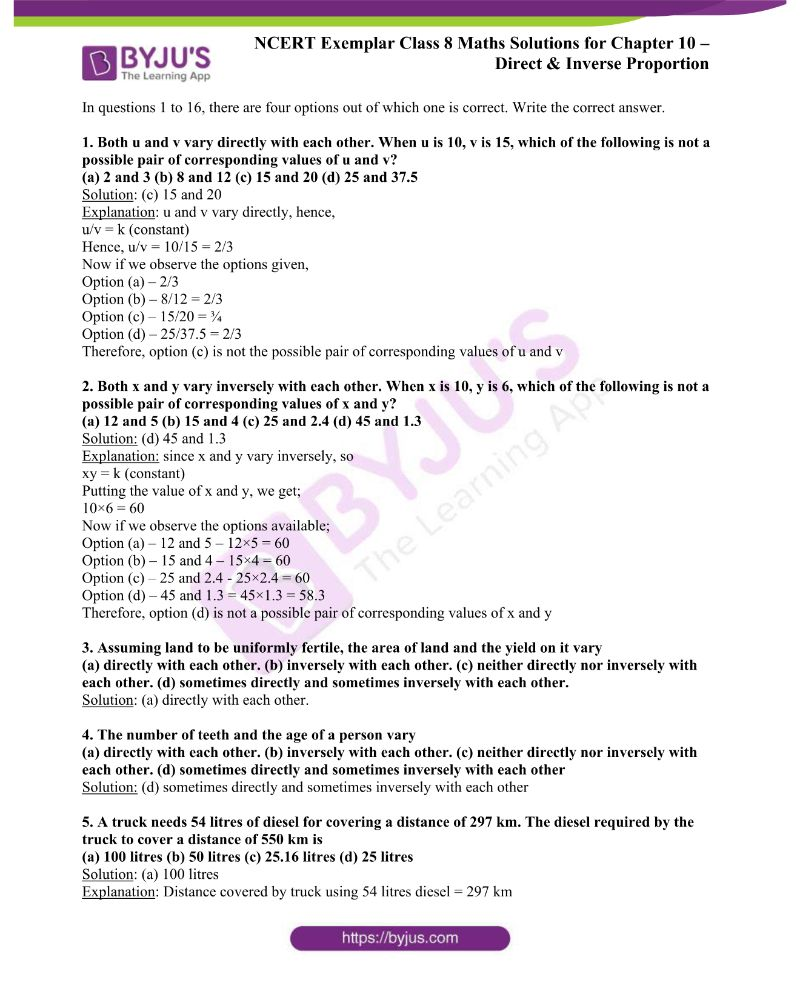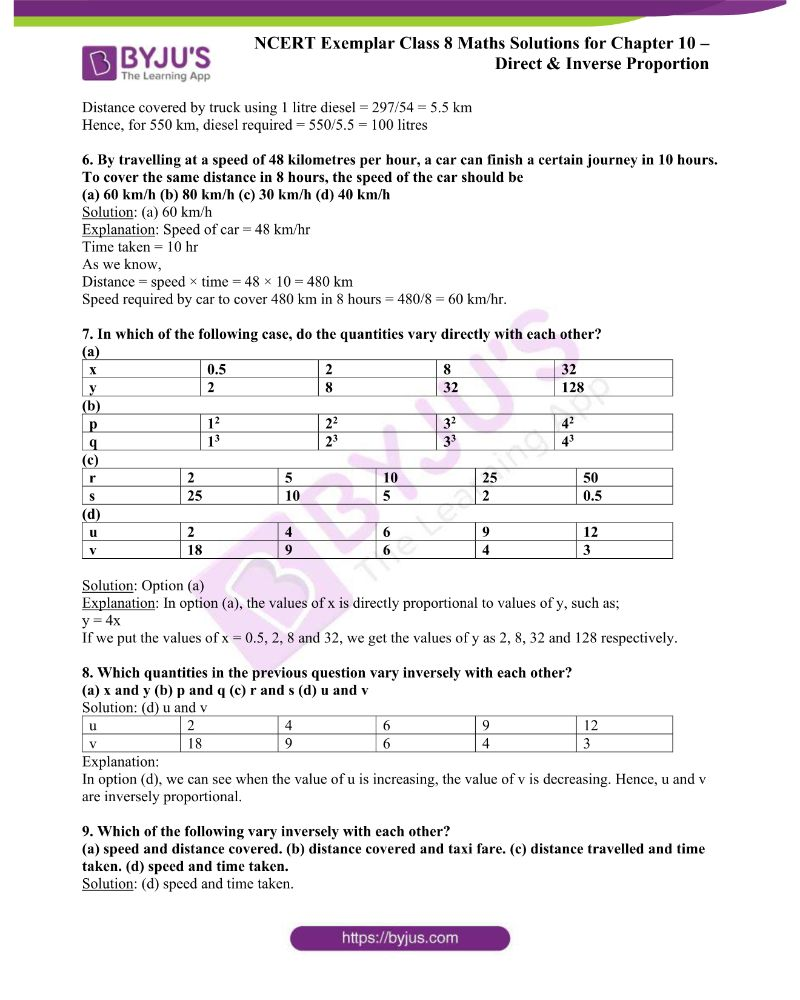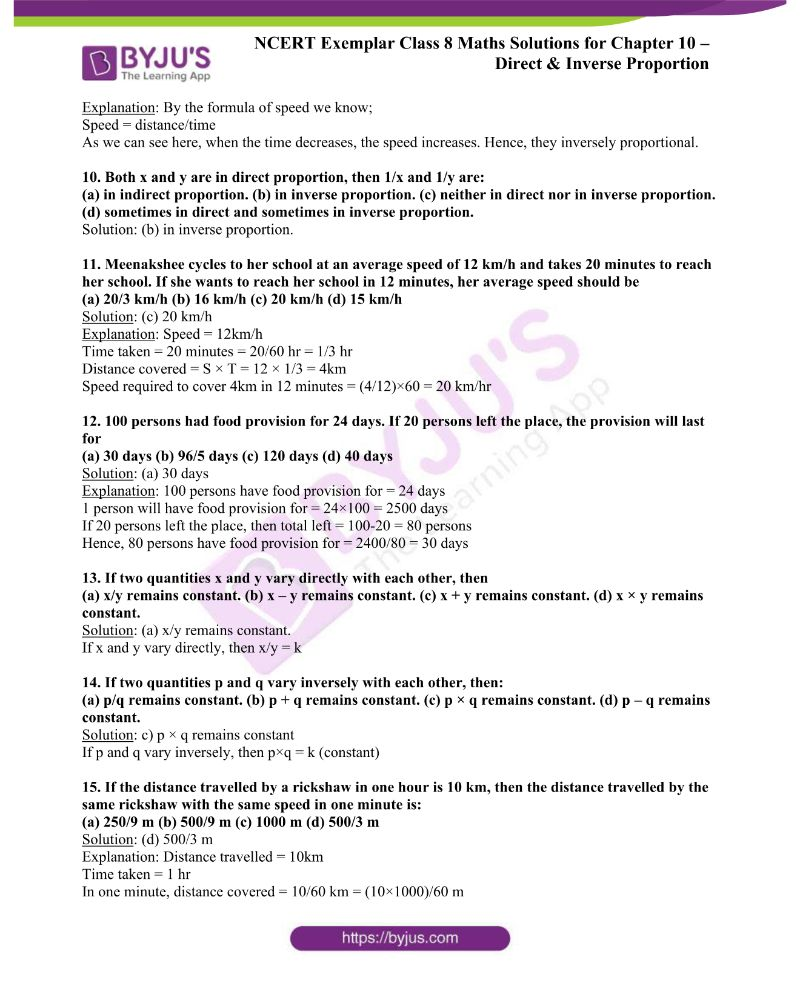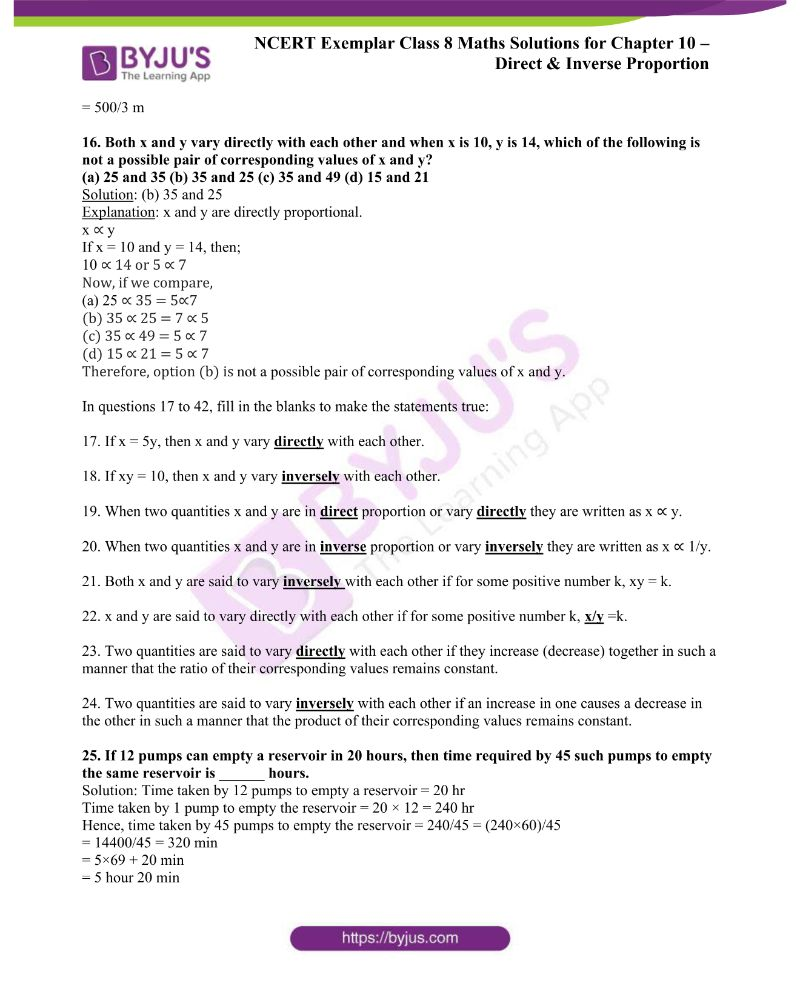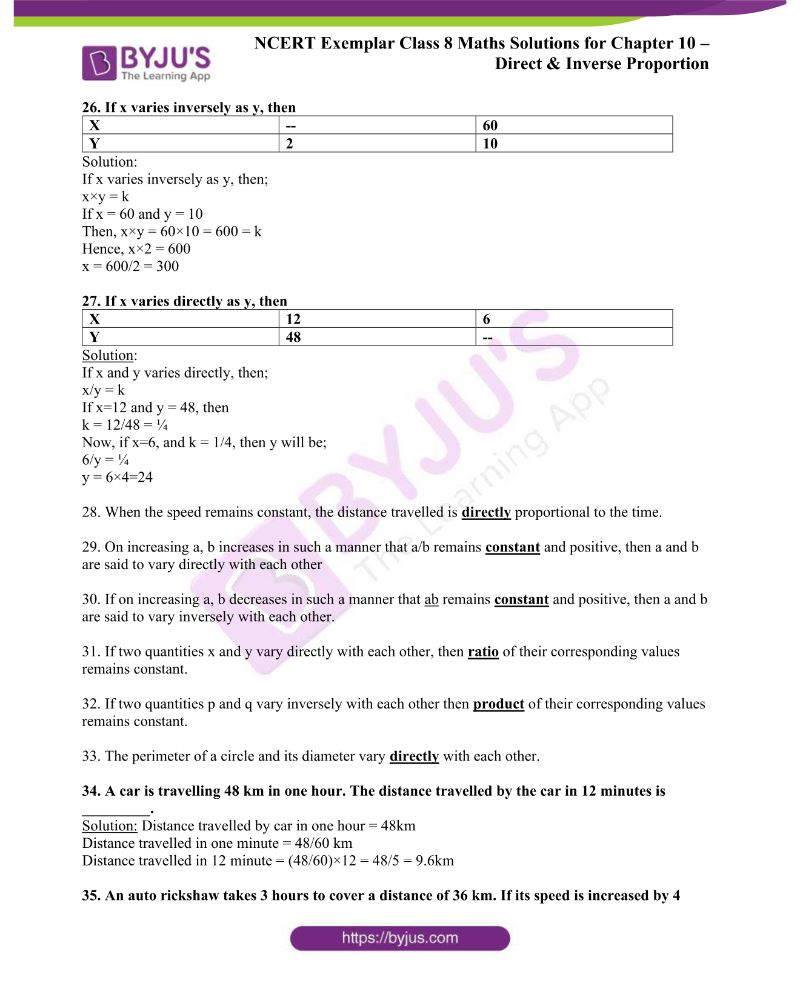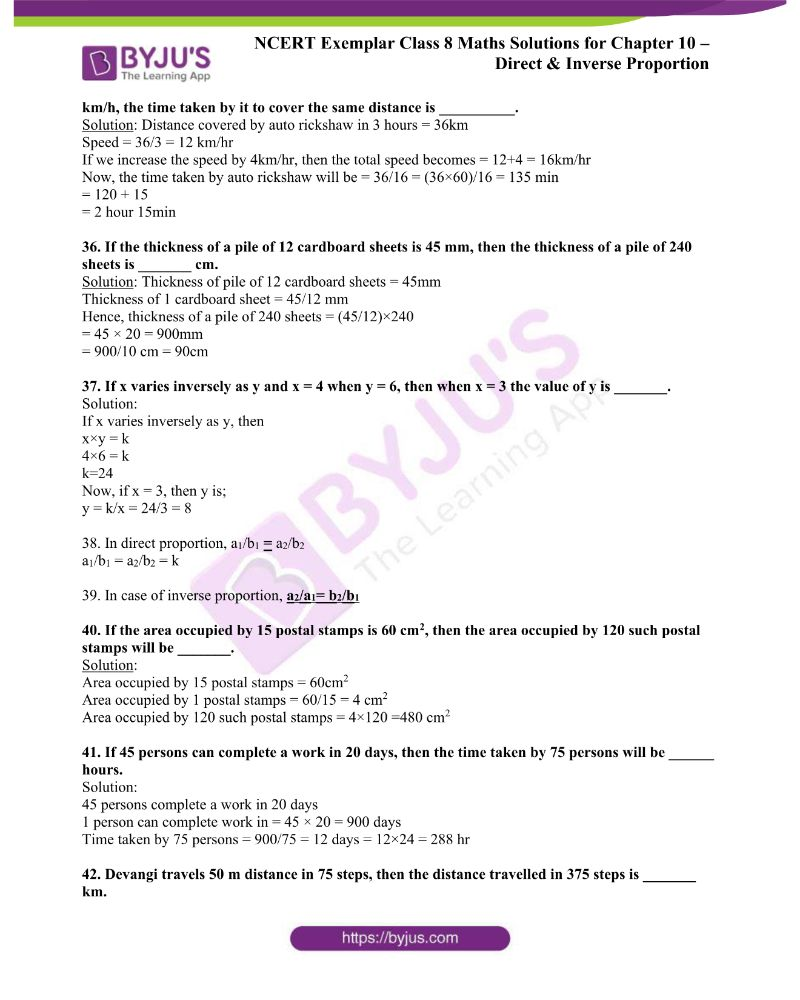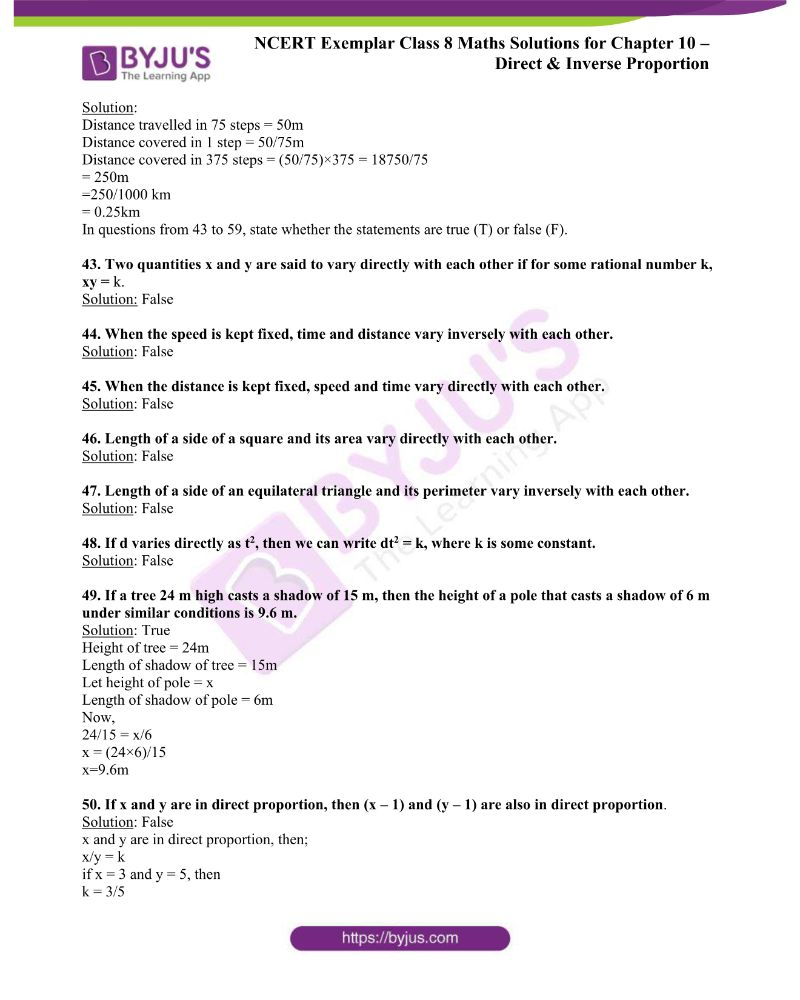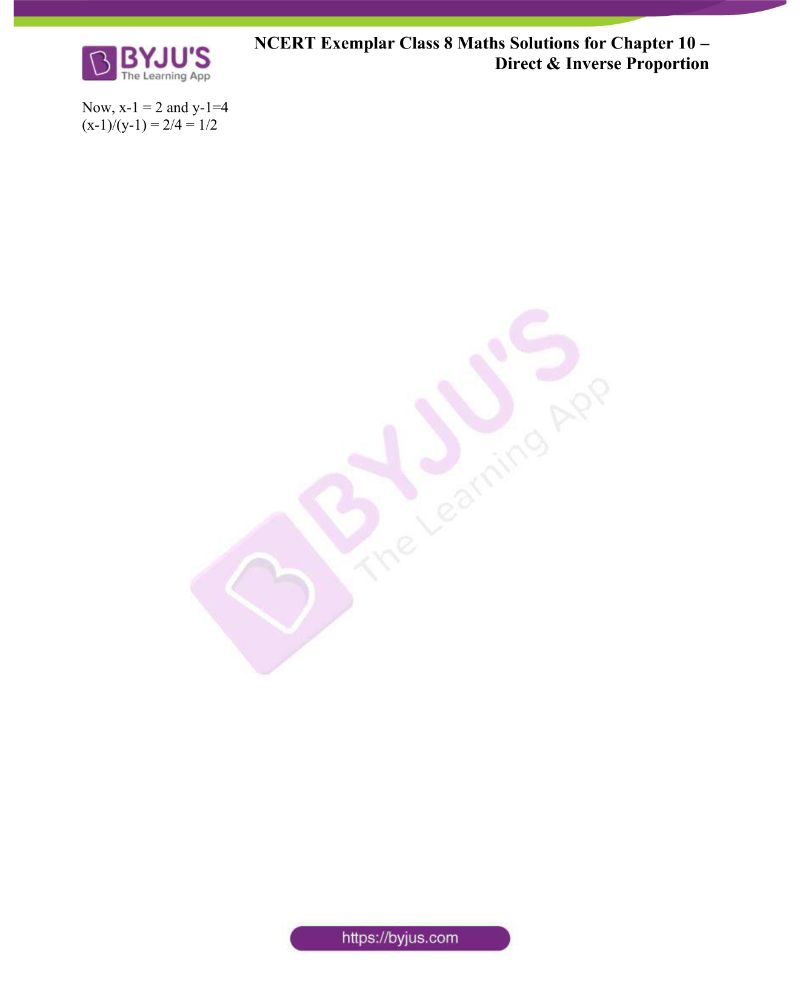### Access NCERT Exemplar Solutions for Class 8 Maths Chapter 10 Direct and Inverse Proportions

In questions 1 to 16, there are four options, out of which one is correct. Write the correct answer.

1. Both u and v vary directly with each other. When u is 10, and v is 15, which of the following is not a possible pair of corresponding values of u and v?

(a) 2 and 3 (b) 8 and 12 (c) 15 and 20 (d) 25 and 37.5

Solution: (c) 15 and 20

Explanation: u and v vary directly, hence,

u/v = k (constant)

Hence, u/v = 10/15 = 2/3

Now if we observe the options given,

Option (a) – 2/3

Option (b) – 8/12 = 2/3

Option (c) – 15/20 = ¾

Option (d) – 25/37.5 = 2/3

Therefore, option (c) is not the possible pair of corresponding values of u and v

2. Both x and y vary inversely with each other. When x is 10, and y is 6, which of the following is not a possible pair of corresponding values of x and y?

(a) 12 and 5 (b) 15 and 4 (c) 25 and 2.4 (d) 45 and 1.3

Solution: (d) 45 and 1.3

Explanation: since x and y vary inversely, so

xy = k (constant)

Putting the value of x and y, we get;

10×6 = 60

Now if we observe the options available;

Option (a) – 12 and 5 – 12×5 = 60

Option (b) – 15 and 4 – 15×4 = 60

Option (c) – 25 and 2.4 – 25×2.4 = 60

Option (d) – 45 and 1.3 = 45×1.3 = 58.3

Therefore, option (d) is not a possible pair of corresponding values of x and y

3. Assuming land to be uniformly fertile, the area of land and the yield on it vary

(a) directly with each other. (b) inversely with each other. (c) neither directly nor inversely with each other. (d) sometimes directly and sometimes inversely with each other.

Solution: (a) directly with each other.

4. The number of teeth and the age of a person vary

(a) directly with each other. (b) inversely with each other. (c) neither directly nor inversely with each other. (d) sometimes directly and sometimes inversely with each other

Solution: (d) sometimes directly and sometimes inversely with each other

5. A truck needs 54 litres of diesel to cover a distance of 297 km. The diesel required by the truck to cover a distance of 550 km is

(a) 100 litres (b) 50 litres (c) 25.16 litres (d) 25 litres

Solution: (a) 100 litres

Explanation: Distance covered by the truck using 54 litres of diesel = 297 km

Distance covered by truck using 1 litre diesel = 297/54 = 5.5 km

Hence, for 550 km, diesel required = 550/5.5 = 100 litres

6. By travelling at a speed of 48 kilometres per hour, a car can finish a certain journey in 10 hours. To cover the same distance in 8 hours, the speed of the car should be

(a) 60 km/h (b) 80 km/h (c) 30 km/h (d) 40 km/h

Solution: (a) 60 km/h

Explanation: Speed of car = 48 km/hr

Time taken = 10 hr

As we know,

Distance = speed × time = 48 × 10 = 480 km

The speed required by a car to cover 480 km in 8 hours = 480/8 = 60 km/hr.

7. In which of the following case do the quantities vary directly with each other?

(a)

 x 0.5 2 8 32 y 2 8 32 128

(b)

 p 12 22 32 42 q 13 23 33 43

(c)

 r 2 5 10 25 50 s 25 10 5 2 0.5

(d)

 u 2 4 6 9 12 v 18 9 6 4 3

Solution: Option (a)

Explanation: In option (a), the values of x are directly proportional to values of y, such as;

y = 4x

If we put the values of x = 0.5, 2, 8 and 32, we get the values of y as 2, 8, 32 and 128, respectively.

8. Which quantities in the previous question vary inversely with each other?

(a) x and y (b) p and q (c) r and s (d) u and v

Solution: (d) u and v

 u 2 4 6 9 12 v 18 9 6 4 3

Explanation:

In option (d), we can see when the value of u increases, the value of v decreases. Hence, u and v are inversely proportional.

9. Which of the following vary inversely with each other?

(a) speed and distance covered. (b) distance covered and taxi fare. (c) distance travelled and time taken. (d) speed and time taken.

Solution: (d) speed and time taken.

Explanation: By the formula of speed, we know;

Speed = distance/time

As we can see here, when the time decreases, the speed increases. Hence, they are inversely proportional.

10. Both x and y are in direct proportion, then 1/x and 1/y are:

(a) in indirect proportion. (b) in inverse proportion. (c) neither in direct nor in inverse proportion. (d) sometimes in direct and sometimes in inverse proportion.

Solution: (b) in inverse proportion.

11. Meenakshee cycles to her school at an average speed of 12 km/h and takes 20 minutes to reach her school. If she wants to reach her school in 12 minutes, her average speed should be

(a) 20/3 km/h (b) 16 km/h (c) 20 km/h (d) 15 km/h

Solution: (c) 20 km/h

Explanation: Speed = 12km/h

Time taken = 20 minutes = 20/60 hr = 1/3 hr

Distance covered = S × T = 12 × 1/3 = 4km

Speed required to cover 4km in 12 minutes = (4/12)×60 = 20 km/hr

12. 100 persons had food provision for 24 days. If 20 persons left the place, the provision will last for

(a) 30 days (b) 96/5 days (c) 120 days (d) 40 days

Solution: (a) 30 days

Explanation: 100 persons have food provision for = 24 days

1 person will have food provision for = 24×100 = 2500 days

If 20 persons left the place, then the total left = 100-20 = 80 persons

Hence, 80 persons have food provision for = 2400/80 = 30 days

13. If two quantities, x and y, vary directly with each other, then

(a) x/y remains constant. (b) x – y remains constant. (c) x + y remains constant. (d) x × y remains constant.

Solution: (a) x/y remains constant.

If x and y vary directly, then x/y = k

14. If two quantities p and q vary inversely with each other, then:

(a) p/q remains constant. (b) p + q remains constant. (c) p × q remains constant. (d) p – q remains constant.

Solution: c) p × q remains constant

If p and q vary inversely, then p×q = k (constant)

15. If the distance travelled by a rickshaw in one hour is 10 km, then the distance travelled by the same rickshaw with the same speed in one minute is:

(a) 250/9 m (b) 500/9 m (c) 1000 m (d) 500/3 m

Solution: (d) 500/3 m

Explanation: Distance travelled = 10km

Time taken = 1 hr

In one minute, distance covered = 10/60 km = (10×1000)/60 m

= 500/3 m

16. Both x and y vary directly with each other, and when x is 10, y is 14, which of the following is not a possible pair of corresponding values of x and y?

(a) 25 and 35 (b) 35 and 25 (c) 35 and 49 (d) 15 and 21

Solution: (b) 35 and 25

Explanation: x and y are directly proportional.

x ∝ y

If x = 10 and y = 14, then;

10 ∝ 14 or 5 ∝ 7

Now, if we compare,

(a) 25 ∝ 35 = 5∝7

(b) 35 ∝ 25 = 7 ∝ 5

(c) 35 ∝ 49 = 5 ∝ 7

(d) 15 ∝ 21 = 5 ∝ 7

Therefore, option (b) is not a possible pair of corresponding values of x and y.

In questions 17 to 42, fill in the blanks to make the statements true:

17. If x = 5y, then x and y vary directly with each other.

18. If xy = 10, then x and y vary inversely with each other.

19. When two quantities, x and y are in direct proportion or vary directly, they are written as x ∝ y.

20. When two quantities, x and y are in inverse proportion or vary inversely, they are written as x ∝ 1/y.

21. Both x and y are said to vary inversely with each other if, for some positive number k, xy = k.

22. x and y are said to vary directly with each other if, for some positive number k, x/y =k.

23. Two quantities are said to vary directly with each other if they increase (decrease) together in such a manner that the ratio of their corresponding values remains constant.

24. Two quantities are said to vary inversely with each other if an increase in one causes a decrease in the other in such a manner that the product of their corresponding values remains constant.

25. If 12 pumps can empty a reservoir in 20 hours, then the time required by 45 such pumps to empty the same reservoir is ______ hours.

Solution: Time taken by 12 pumps to empty a reservoir = 20 hr

Time taken by 1 pump to empty the reservoir = 20 × 12 = 240 hr

Hence, time taken by 45 pumps to empty the reservoir = 240/45 = (240×60)/45

= 14400/45 = 320 min

= 5×69 + 20 min

= 5 hours 20 min

26. If x varies inversely as y, then

 X — 60 Y 2 10

Solution:

If x varies inversely as y, then;

x×y = k

If x = 60 and y = 10

Then, x×y = 60×10 = 600 = k

Hence, x×2 = 600

x = 600/2 = 300

27. If x varies directly as y, then

 X 12 6 Y 48 —

Solution:

If x and y vary directly, then;

x/y = k

If x=12 and y = 48, then

k = 12/48 = ¼

Now, if x=6, and k = 1/4, then y will be;

6/y = ¼

y = 6×4=24

28. When the speed remains constant, the distance travelled is directly proportional to the time.

29. On increasing a, b increases in such a manner that a/b remains constant and positive, then a and b are said to vary directly with each other

30. If on increasing a, b decreases in such a manner that ab remains constant and positive, then a and b are said to vary inversely with each other.

31. If two quantities, x and y vary directly with each other, then the ratio of their corresponding values remains constant.

32. If two quantities, p and q vary inversely with each other, then the product of their corresponding values remains constant.

33. The perimeter of a circle and its diameter vary directly with each other.

34. A car is travelling 48 km in one hour. The distance travelled by the car in 12 minutes is _________.

Solution: Distance travelled by car in one hour = 48km

Distance travelled in one minute = 48/60 km

Distance travelled in 12 minute = (48/60)×12 = 48/5 = 9.6km

35. An auto rickshaw takes 3 hours to cover a distance of 36 km. If its speed is increased by 4 km/h, the time taken by it to cover the same distance is __________.

Solution: Distance covered by auto rickshaw in 3 hours = 36km

Speed = 36/3 = 12 km/hr

If we increase the speed by 4km/hr, then the total speed becomes = 12+4 = 16km/hr

Now, the time taken by auto rickshaw will be = 36/16 = (36×60)/16 = 135 min

= 120 + 15

= 2 hours 15min

36. If the thickness of a pile of 12 cardboard sheets is 45 mm, then the thickness of a pile of 240 sheets is _______ cm.

Solution: Thickness of pile of 12 cardboard sheets = 45mm

The thickness of 1 cardboard sheet = 45/12 mm

Hence, the thickness of a pile of 240 sheets = (45/12)×240

= 45 × 20 = 900mm

= 900/10 cm = 90cm

37. If x varies inversely as y and x = 4 when y = 6, then when x = 3 the value of y is _______.

Solution:

If x varies inversely as y, then

x×y = k

4×6 = k

k=24

Now, if x = 3, then y is;

y = k/x = 24/3 = 8

38. In direct proportion, a1/b1 = a2/b2

a1/b1 = a2/b2 = k

39. In case of inverse proportion, a2/a1= b2/b1

40. If the area occupied by 15 postal stamps is 60 cm2, then the area occupied by 120 such postal stamps will be _______.

Solution:

The area occupied by 15 postal stamps = 60cm2

Area occupied by 1 postal stamps = 60/15 = 4 cm2

The area occupied by 120 such postal stamps = 4×120 =480 cm2

41. If 45 persons can complete a work in 20 days, then the time taken by 75 persons will be ______ hours.

Solution:

45 persons complete a work in 20 days

1 person can complete work in = 45 × 20 = 900 days

Time taken by 75 persons = 900/75 = 12 days = 12×24 = 288 hr

42. Devangi travels 50 m distance in 75 steps, then the distance travelled in 375 steps is _______ km.

Solution:

Distance travelled in 75 steps = 50m

Distance covered in 1 step = 50/75m

Distance covered in 375 steps = (50/75)×375 = 18750/75

= 250m

=250/1000 km

= 0.25km

In questions from 43 to 59, state whether the statements are true (T) or false (F).

43. Two quantities, x and y, are said to vary directly with each other if, for some rational number k, xy = k.

Solution: False

44. When the speed is kept fixed, time and distance vary inversely with each other.

Solution: False

45. When the distance is kept fixed, speed and time vary directly with each other.

Solution: False

46. Length of a side of a square and its area varies directly with each other.

Solution: False

47. Length of a side of an equilateral triangle and its perimeter vary inversely with each other.

Solution: False

48. If d varies directly as t2, then we can write dt2 = k, where k is some constant.

Solution: False

49. If a tree 24 m high casts a shadow of 15 m, then the height of a pole that casts a shadow of 6 m under similar conditions is 9.6 m.

Solution: True

Height of tree = 24m

Length of the shadow of the tree = 15m

Let the height of the pole = x

Length of the shadow of the pole = 6m

Now,

24/15 = x/6

x = (24×6)/15

x=9.6m

50. If x and y are in direct proportion, then (x – 1) and (y – 1) are also in direct proportion.

Solution: False

x and y are in direct proportion, then;

x/y = k

if x = 3 and y = 5, then

k = 3/5

Now, x-1 = 2 and y-1=4

(x-1)/(y-1) = 2/4 = 1/2

Solve Class 8 Maths Exemplar Solutions for all chapters and understand the concepts in an easy way. Also, download BYJU’S – The Learning App to get personalised video content and experience a new way of learning to understand the concepts easily.

## Frequently Asked Questions on NCERT Exemplar Solutions for Class 8 Maths Chapter 10

Q1

### How is NCERT Exemplar Solutions for Class 8 Maths Chapter 10 helpful for Class 8 students?

NCERT Exemplar Solutions for Class 8 Maths Chapter 10 are helpful for students to get a proper grasp of all the concepts covered in this chapter and also to lay the foundation for their higher studies. BYJU’S experts formulated these solutions in an easy-to-understandable manner that will help students solve problems in the most efficient ways. Also, these solutions will help CBSE Class 8 students to build a strong foundation of the concepts and secure excellent marks in their final exams.
Q2

### Why should we follow NCERT Exemplar Solutions for Class 8 Maths Chapter 10?

NCERT Exemplar Solutions for Class 8 Maths Chapter 10 is the proper learning resource that is devised to help students master the concepts. Revising the chapter using the exemplar solutions, along with the textbooks, will help them crack different types of questions asked in the annual exam, as well as boost their problem-solving and logical reasoning skills.  Hence, they are the most popular study materials used by students to prepare for the CBSE annual exams. Practising these solutions will help them to score good marks in the final exams.
Q3

### Where to download NCERT Exemplar Solutions for Class 8 Maths Chapter 10?

NCERT Exemplar Solutions for Class 8 Maths Chapter 10 can be downloaded by the students in PDF for offline use or can be referred to online on BYJU’S website. These solutions are formulated by expert faculty at BYJU’S according to the latest CBSE syllabus, covering the questions in the NCERT Exemplar textbook of Class 8 Maths.